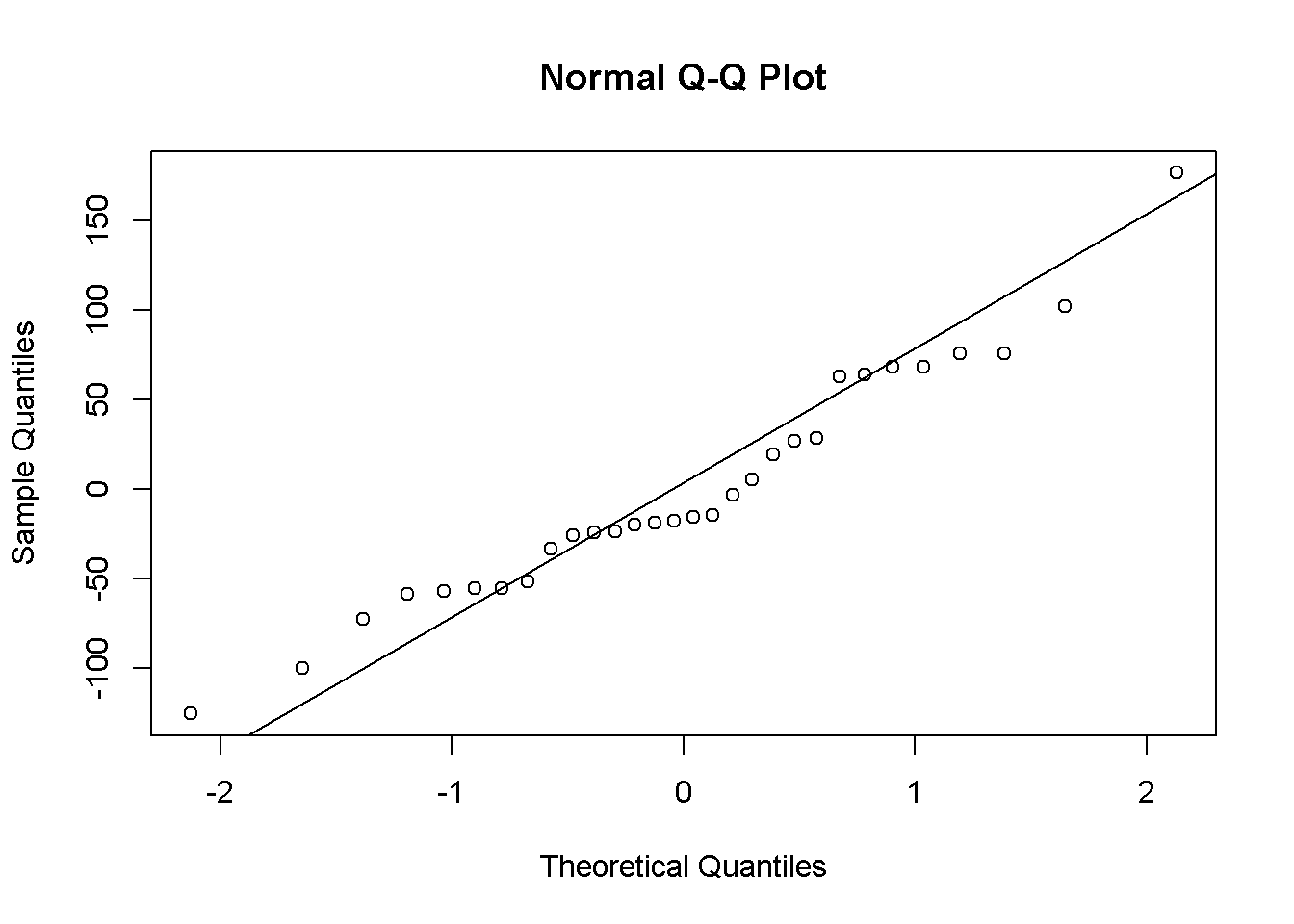download.file("http://www.openintro.org/stat/data/mlb11.RData", destfile = "mlb11.RData")
load("mlb11.RData")

#### Exercise 1. What type of plot would you use to display the relationship between runs and one of the other numerical variables? Plot this relationship using the variable at_bats as the predictor. Does the relationship look linear? If you knew a team’s at_bats, would you be comfortable using a linear model to predict the number of runs?

##### Use scatterplot to display relationship between runs and at_bats. There is a positive correlation and roughly linear, I would be comfortable using a linear model to predict number of runs.
plot(mlb11$at_bats,mlb11$runs)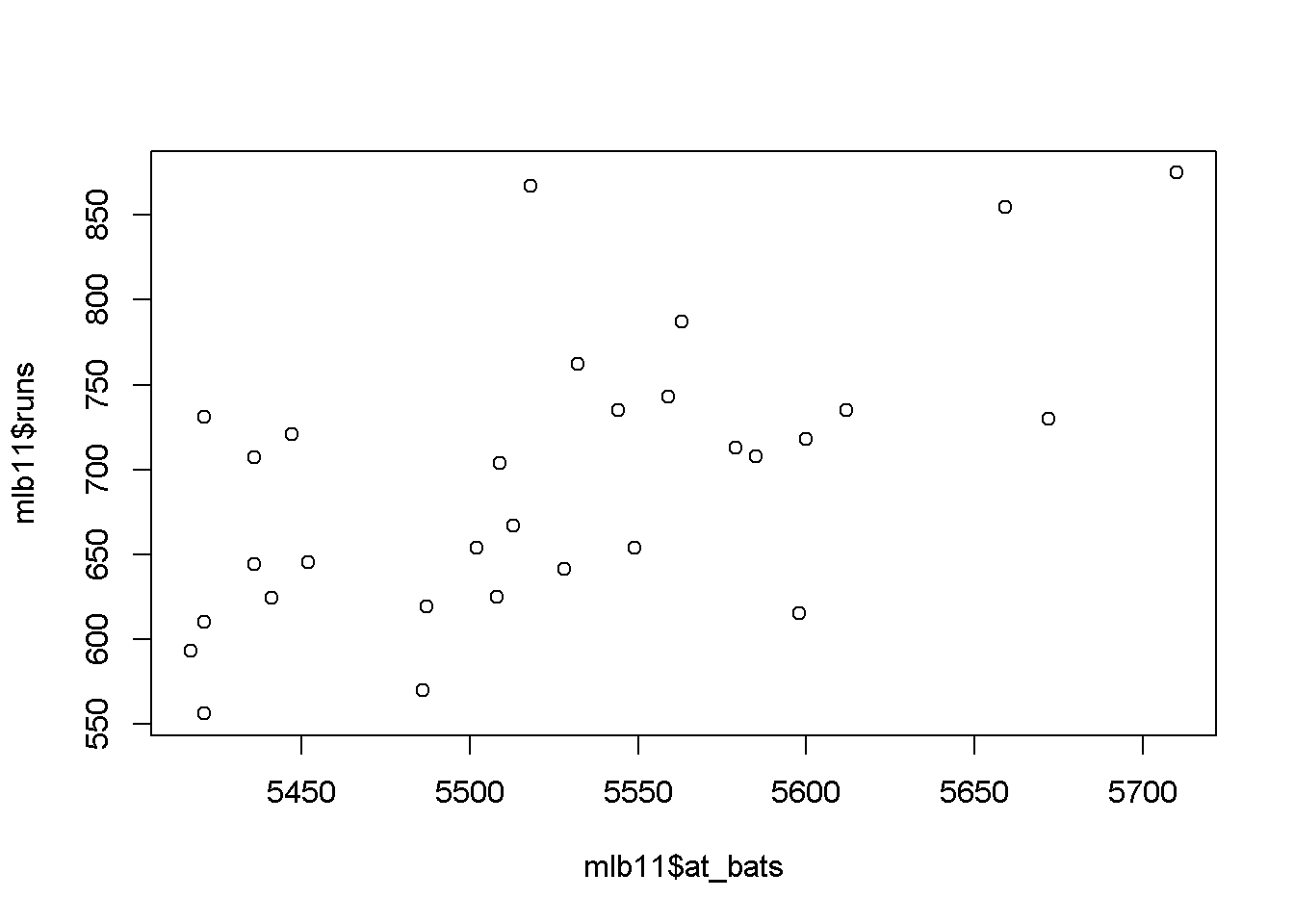cor(mlb11$runs, mlb11$at_bats)
##  0.610627

### Sum of squared residuals

#### Exercise 2.Looking at your plot from the previous exercise, describe the relationship between these two variables. Make sure to discuss the form, direction, and strength of the relationship as well as any unusual observations.

##### The plot has positive correlation between at_bats and runs. There are a few outliers but the scatterplot does not show any curves, thus the relationship between 2 variables is roughly linear.
plot_ss(x = mlb11$at_bats, y = mlb11$runs)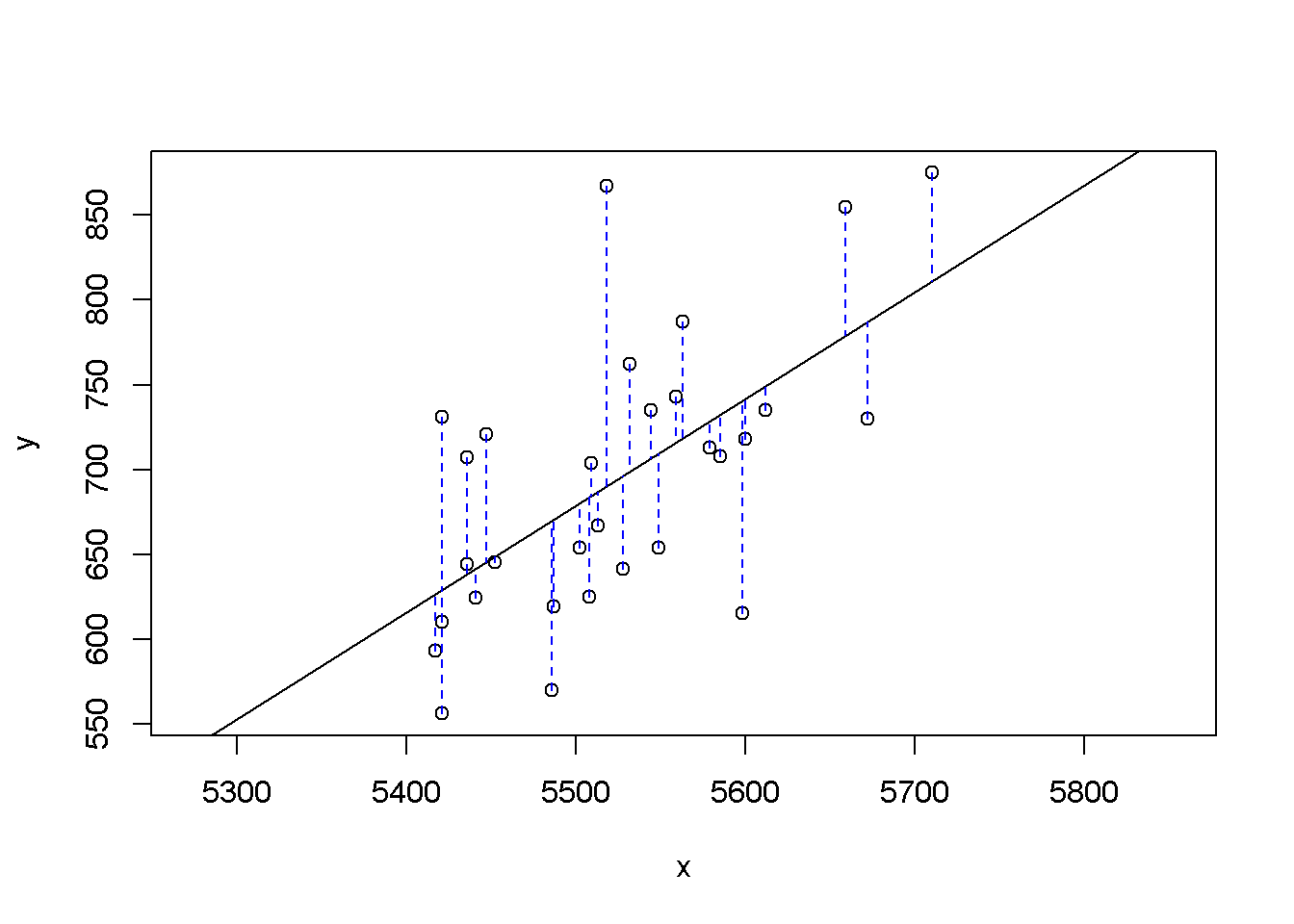## Click two points to make a line.

## Call:
## lm(formula = y ~ x, data = pts)
##
## Coefficients:
## (Intercept)            x
##  -2789.2429       0.6305
##
## Sum of Squares:  123721.9
plot_ss(x = mlb11$at_bats, y = mlb11$runs, showSquares = TRUE)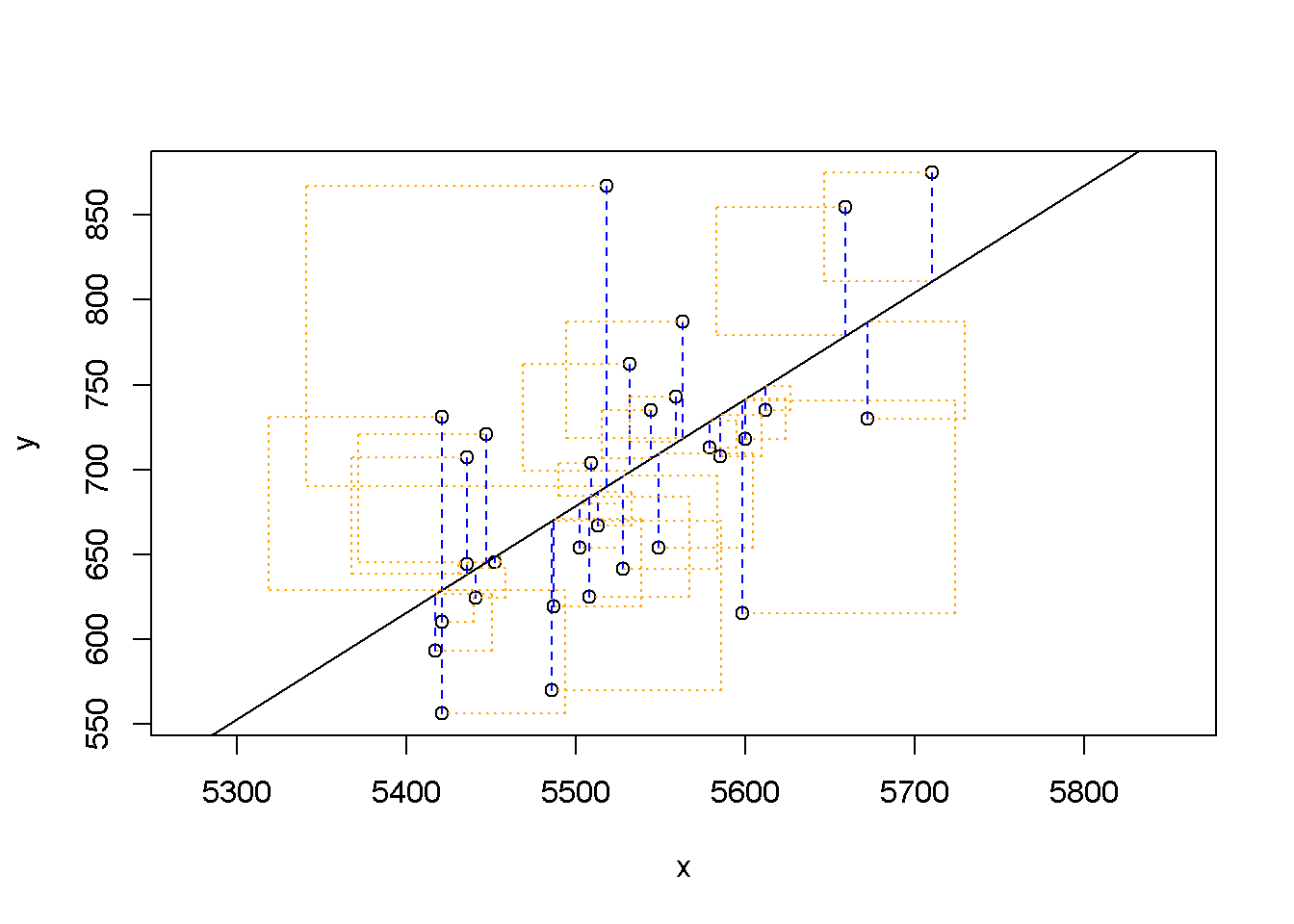## Click two points to make a line.

## Call:
## lm(formula = y ~ x, data = pts)
##
## Coefficients:
## (Intercept)            x
##  -2789.2429       0.6305
##
## Sum of Squares:  123721.9

### The linear model

m1 <- lm(runs ~ at_bats, data = mlb11)
summary(m1)
##
## Call:
## lm(formula = runs ~ at_bats, data = mlb11)
##
## Residuals:
##     Min      1Q  Median      3Q     Max
## -125.58  -47.05  -16.59   54.40  176.87
##
## Coefficients:
##               Estimate Std. Error t value Pr(>|t|)
## (Intercept) -2789.2429   853.6957  -3.267 0.002871 **
## at_bats         0.6305     0.1545   4.080 0.000339 ***
## ---
## Signif. codes:  0 '***' 0.001 '**' 0.01 '*' 0.05 '.' 0.1 ' ' 1
##
## Residual standard error: 66.47 on 28 degrees of freedom
## Multiple R-squared:  0.3729, Adjusted R-squared:  0.3505
## F-statistic: 16.65 on 1 and 28 DF,  p-value: 0.0003388

#### Excercise 4. Fit a new model that uses homeruns to predict runs. Using the estimates from the R output, write the equation of the regression line. What does the slope tell us in the context of the relationship between success of a team and its home runs?

##### equation of regression line is y=1.8345x + 415.239. The slope indicates that the there is positive relationship between success of a tean and home runs.
m2 <- lm(runs ~ homeruns, data = mlb11)
summary(m2)
##
## Call:
## lm(formula = runs ~ homeruns, data = mlb11)
##
## Residuals:
##     Min      1Q  Median      3Q     Max
## -91.615 -33.410   3.231  24.292 104.631
##
## Coefficients:
##             Estimate Std. Error t value Pr(>|t|)
## (Intercept) 415.2389    41.6779   9.963 1.04e-10 ***
## homeruns      1.8345     0.2677   6.854 1.90e-07 ***
## ---
## Signif. codes:  0 '***' 0.001 '**' 0.01 '*' 0.05 '.' 0.1 ' ' 1
##
## Residual standard error: 51.29 on 28 degrees of freedom
## Multiple R-squared:  0.6266, Adjusted R-squared:  0.6132
## F-statistic: 46.98 on 1 and 28 DF,  p-value: 1.9e-07

### Prediction and prediction errors

plot(mlb11$runs ~ mlb11$at_bats)
abline(m1)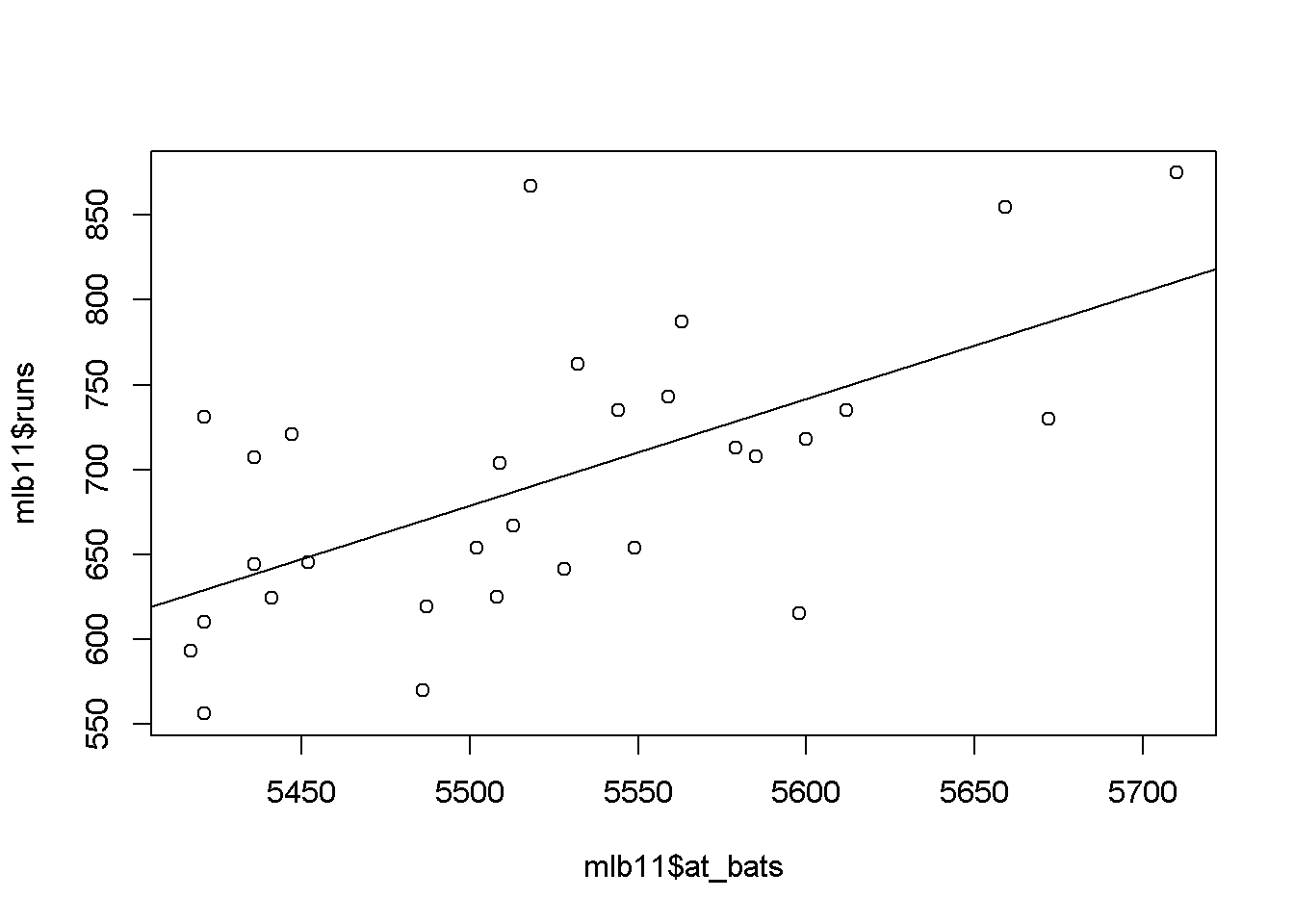m1
##
## Call:
## lm(formula = runs ~ at_bats, data = mlb11)
##
## Coefficients:
## (Intercept)      at_bats
##  -2789.2429       0.6305

#### Exercise 5.If a team manager saw the least squares regression line and not the actual data, how many runs would he or she predict for a team with 5,578 at-bats? Is this an overestimate or an underestimate, and by how much? In other words, what is the residual for this prediction?

##### Manager can substitute x with 5578 and predict number of runs. She would estimate 728 runs. Doing a search in the table, there is a very close number of at_bats of 5579 from the phillies. The total runs were 713. Manager would overestimate by 14 runs.

###Model diagnostics

plot(m1$residuals ~ mlb11$at_bats)
abline(h = 0, lty = 3)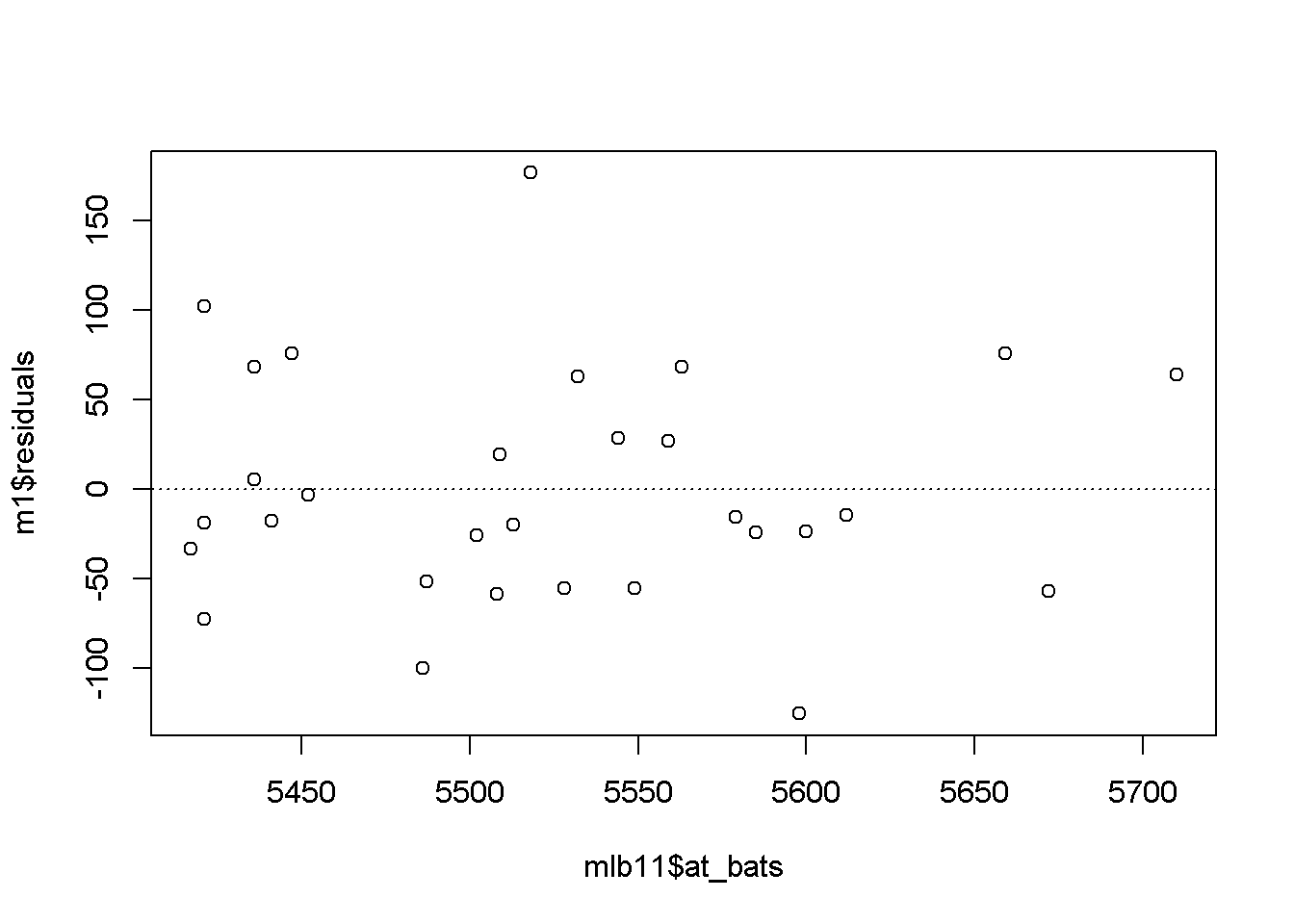#### Exercise 6. Is there any apparent pattern in the residuals plot? What does this indicate about the linearity of the relationship between runs and at-bats?

##### there is no patter in the residual plot. This indicate that there is a linear relationship between runs and at_bats.
hist(m1$residuals)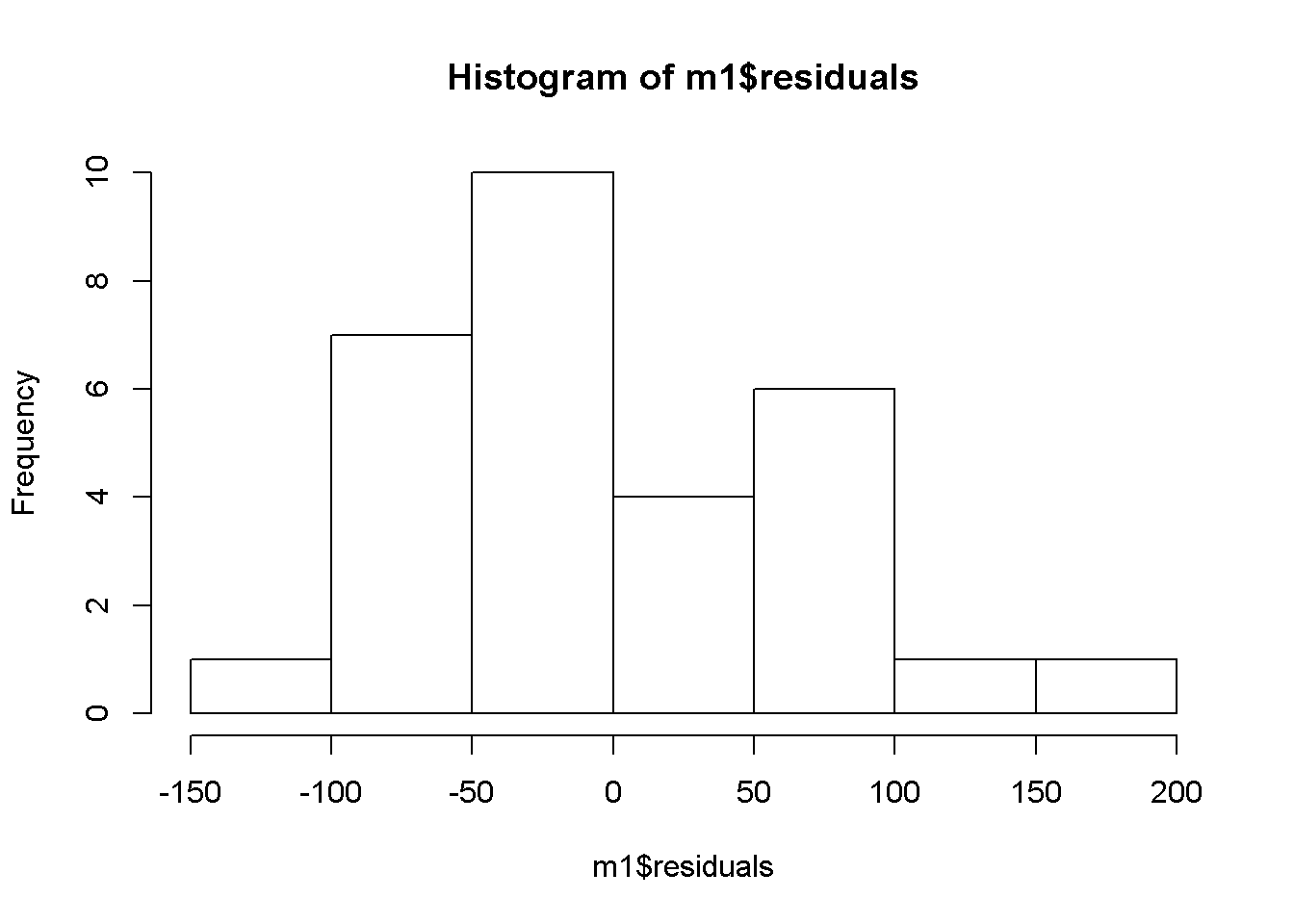qqnorm(m1$residuals)
qqline(m1\$residuals)